# Ampere’s Circuital Law: What is it?

Ampere’s Circuital Law states the relationship between the current and the magnetic field created by it.

This law states that the integral of magnetic field density (B) along an imaginary closed path is equal to the product of current enclosed by the path and permeability of the medium.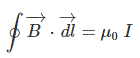James Clerk Maxwell had derived that.
It alternatively says, the integral of magnetic field intensity (H) along an imaginary closed path is equal to the current enclosed by the path.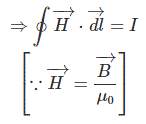Let us take an electrical conductor, carrying a current of I ampere, downward as shown in the figure below.Let us take an imaginary loop around the conductor. We also call this loop as amperian loop.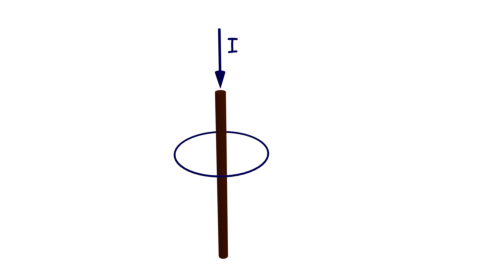Let us also imagine the radius of the loop is r and the flux density created at any point on the loop due to current through the conductor is B.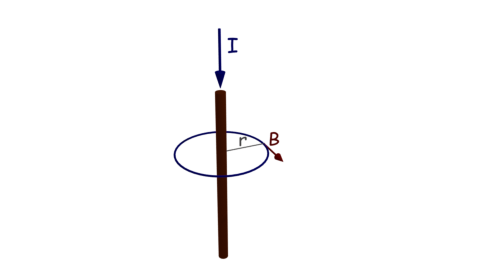Let us consider an infinitesimal length dl of the amperian loop at the same point.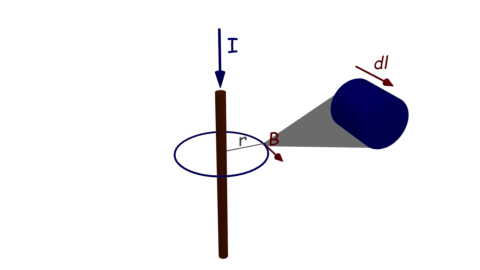At each point on the amperian loop, the value of B is constant since the perpendicular distance of that point from the axis of conductor is fixed, but the direction will be along the tangent on the loop at that point.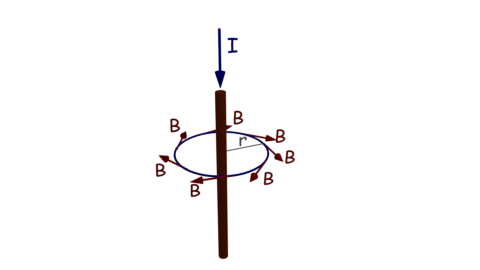The close integral of the magnetic field density B along the amperian loop, will be,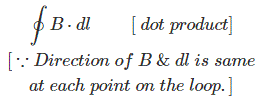Now, according to Ampere’s Circuital Law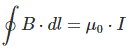Therefore,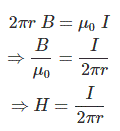Instead of one current carrying conductor, there are N number of conductors carrying same current I, enclosed by the path, then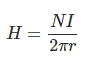Want To Learn Faster? 🎓
Get electrical articles delivered to your inbox every week.
No credit card required—it’s 100% free.Electrical4U is dedicated to the teaching and sharing of all things related to electrical and electronics engineering.

### 2 thoughts on “Ampere’s Circuital Law: What is it?”

1.thx for the help.

•